# AP Statistics : How to interpret histograms

## Example Questions

### Example Question #1 : How To Interpret Histograms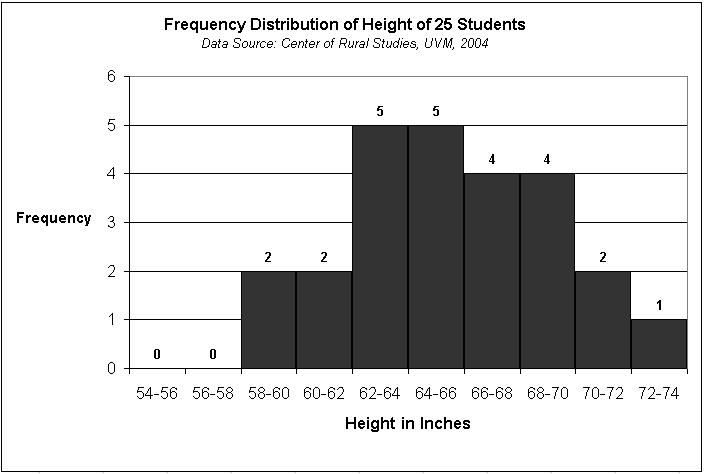Based on the histogram, which set of values was most common in the sample?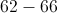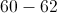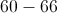Explanation:

Although as a group 62-66 are the most common values in the sample, 60-66 encompasses both 60-62 and 62-66. If you add up the proportion of the sample that is 60-66 inches tall, this proportion is greater than the proportion of the sample that is 60-62 or 62-66 inches tall. Histograms give you the proportion of the sample that has a particular characteristic and you can add up these proportions for a set of characteristics.

### Example Question #1 : How To Interpret Histograms

What is the best measurement of center and spread for the following data set represented by a histogram?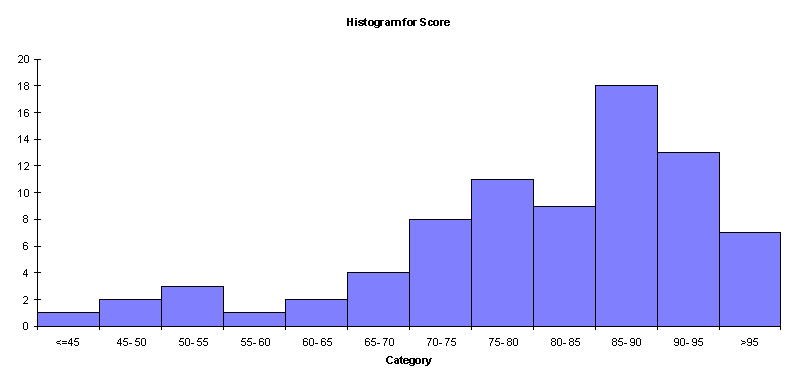Mean and Range

Mean and Standard Deviation

Mean and Interquartile Range

Median and Interquartile Range

Median and Standard Deviation

Median and Interquartile Range

Explanation:

For skewed distributions, the outliers can greatly affect the value of the mean and the standard deviation. Therefore, the median and IQR would be better measures of center and spread because they are not greatly influenced by outlier values.

### Example Question #3 : How To Interpret Histograms

Louie collects data on the amount of sleep students in his class get.

Below is a graphical representation of the data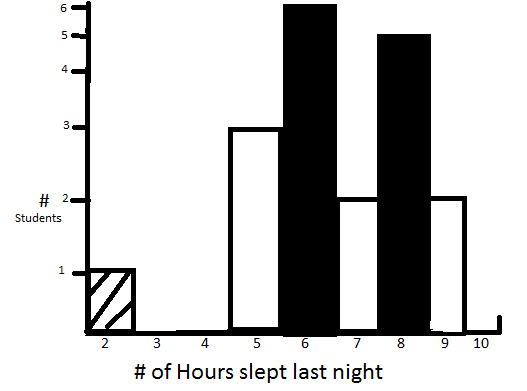Which of the following is/are false about Louie's class' data?

i: the student with 2 hours of sleep is an outlier

ii: without the student with 2 hours of sleep the data is roughly normally distributed

iii: without the student with 2 hours of sleep the data is slightly right skewed

iv: the student with 2 hours of sleep is within 2 standard deviations of the mean

i & ii

iii & iv

ii, iii & iv

i, ii & iii

ii & iv

ii & iv

Explanation:

Entering the data into a calculator you can quickly find the mean to be 6.5 and the sd to be 1.6 6.5-3.2=3.3 3.3>2 so iv is false and by virtue of this 2 is an outlier so i is true.

Between ii and iii, the data is not bell shaped, and does exhibit a slight right skew without the mentioned pt due to more density on 5 and 6 hours than 8 and 9

### Example Question #4 : How To Interpret Histograms

Louie collected the following data on sleeping habits of his classmates:Calculate the median, 1st and 3rd quartile (Q1 and Q3) and mode for the data. There are 19 students in the class.

Median:7     Q1:6       Q3:8        Mode:6

Median:6     Q1:5       Q3:8        Mode:6

Median:7     Q1:6       Q3:9        Mode:8

Median:6     Q1:6       Q3:8        Mode:6

Median:6     Q1:5       Q3:9        Mode:8